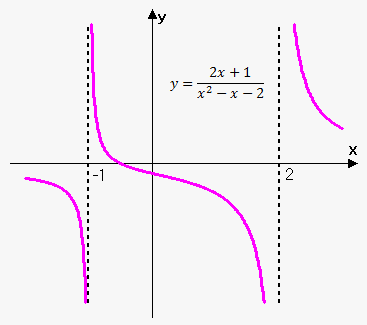# Rational Function

Function of the form f(x)=(Q(x))/(P(x))=(a_0x^n+a_1x^(n-1)+...+a_(n-1)x+a_n)/(b_0x^m+b_1x^(m-1)+...+b_(m-1)x+b_m), where Q(x) and P(x) are polynomials is called rational function.

Domain of this function consists of all x such that Q(x)!=0.Simple example of the rational function is f(x)=(2x+1)/(x^2-x-2)=(2x+1)/((x-2)(x+1)). Its domain is all x except x=2 and x=-1.

This function is shown on the figure.